Ubuntu

# Sort command in Linux with examplesThe sort command is one of the GNU core utilities that is used to sort content in a particular order. It can sort the content in a number of ways that include alphabetically, numerically, by month, in reverse order etc. Here are some key points you need to keep in mind regarding the sort command:

• Digits appear first before letters
• For each letter, lowercase are sorted first.
• By default, spaces are used as a field separator. Other field separators can be used as well.

In this post, we will be showing you the basic usage and some common options of the sort command in Linux. We will be testing all the commands on Ubuntu 20.04 LTS. However, you can apply the same commands on other Linux distributions.

The syntax of the sort command is as follows:

`\$ sort [OPTION]... [FILE]...`

## Basic Usage of the Sort Command

Using the sort command without any command-line options sorts the content of a specified file alphabetically.

Below is our sample1.txt file that we will be using as an example.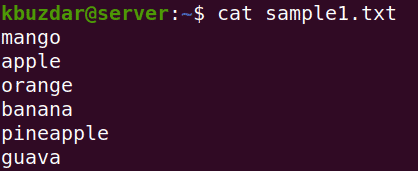To sort the content of the sample1.txt file, the command would be:

`\$ sort sample1.txt`

Here is the output of the above command which shows the alphabetically sorted content.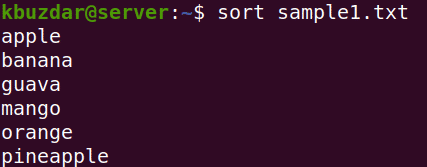If the file contains both alphabets and numbers, then the sort command will sort numbers before alphabets. Below is our sample file which contains both numbers and alphabets: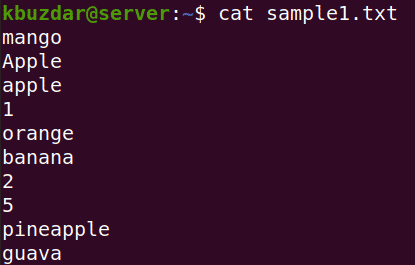Here is the output of the above file after sorting where you can see the numbers are sorted before the alphabets.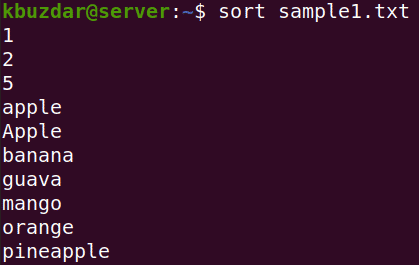## Sort Command Options

The sort command provides a lot of options that expand its usefulness. Let’s have a look at few of the options and how to use them.

### Sort in Reverse Order

To sort the file content in reverse order, use the -r option. For example, below is the content of our sample file which we want to sort in reverse alphabetical order.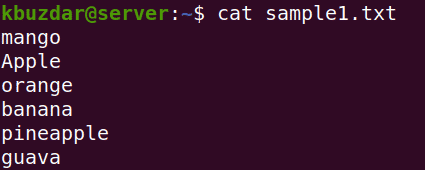To sort the content of this file in reverse order, use the -r option as follows:

`\$ sort -r sample1.txt`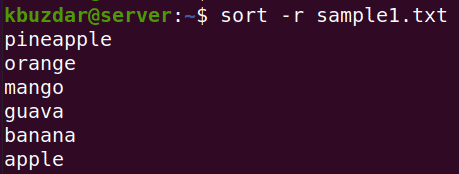### Sort in Numeric Order

To sort the data numerically, use the -n option with the sort command. This option is useful if your file contains numbers and you want to sort them from lowest number to highest or from highest to lowest. Keep in mind that in default sorting, 113 would be considered smaller than 2.

Below is an example of a default sorting of our sample file. Keep in mind that in default sorting, sorting is done on the basis of the first number. If the first number is the same, then it sorts on the basis of the second number. Like in the below example, 113 is smaller than 13 and 2.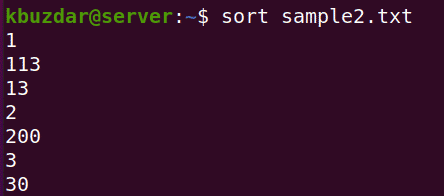To sort the above content numerically (from lowest number to highest), use the -n option as follows:

`\$ sort -n sample2.txt`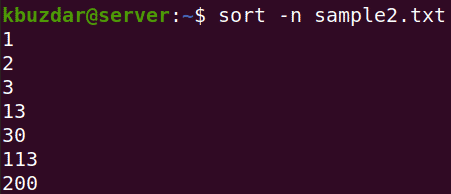### Sort by Month

The default sort command sorts the file content alphabetically. If a file contains a list of month’s names, you can sort them month-wise using the -M command. For example, below is our sample file containing the month names.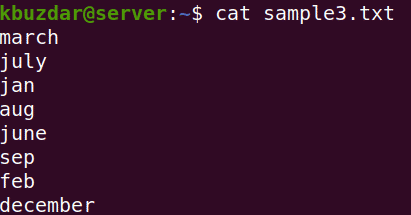To sort the above file content by months, use the -M option as follows:

`\$ sort -M sample3.txt`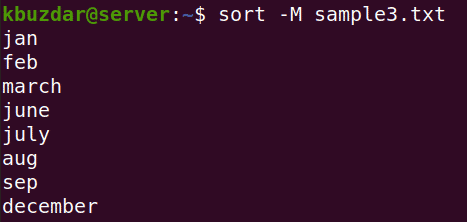### Sort on the Basis of Column Number

By default, the sort command starts sorting the content on the basis of the first character of the first column.

For example, below is our sample file which contains two columns; fruit names and their respective prices. If we use default sorting, it would be done based on the first column (containing fruit names).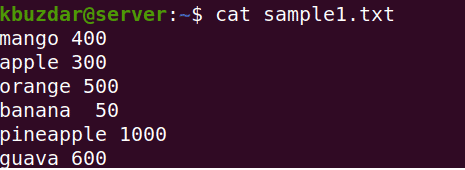To sort the content of the above file on the basis of the second column(prices), we will have to use the -k command as follows:

`\$ sort -k2 -n sample1.txt`

Where -k2 tells the sort command to sort on the second column and -n is used for numerical sort.

The above command will sort the content based on their prices (from lower to higher).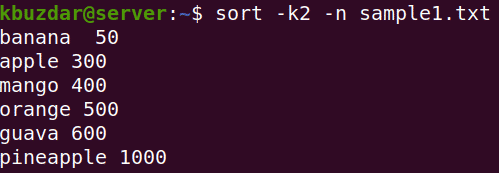### Use Different Field Separator

By default, the sort command uses space as a field separator. To use a different field separator other than space, use the -t option. Below is our sample file that we want to sort on the basis of the second column (separated by commas).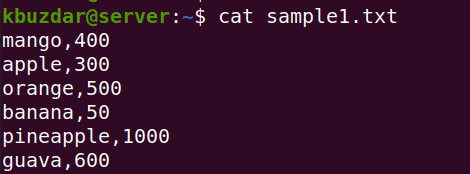The command to sort the above file on the basis of the second column would be:

`\$ sort -k2 -n -t “,” sample1.txt`

Where -k2 tells the sort command to sort on the second column, -n is used for numerical sort and -t is used to specify the field separator.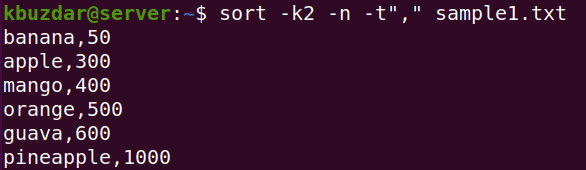### Sort and Remove Duplicates

The -u option with sort command also removes the duplicates along with sorting the content. Below is our sample file which contains the duplicate values.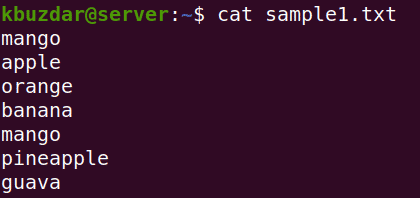To sort this file alphabetically and remove the duplicate values as well, the command would be:

`\$ sort -u sample1.txt`

This is the output of the sorted file with removed duplicates.### Check for Sorted Input

Using the -c option with the sort command, you can check if a file is earlier sorted or not. If a file is earlier sorted, you will see no output. However, if the file is not sorted, it will show the lines which are out of order.

Here is our sample file: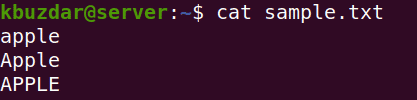To check whether this file is already sorted or not, use the -c option as follows:

`\$ sort -c sample.txt`

As our sample file was already sorted, therefore no output is displayed.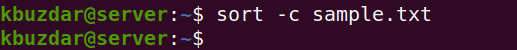### Save Sorted Output in a File

Sort command only displays the sorted output on Terminal; it does not save it. To save the sorted output in a separate file, use the redirect operator. For example, to save the sorted output of file.txt in a new file named sortedfile.txt, use the below command:

`\$ sort file.txt > sortedfile.txt`

### Sort Command using Pipe Option

You can sort the output of other commands by piping them to the sort command. For example, we want to numerically sort a file/directory listing in the Home directory by their sizes. We can do so by using the ls command and piping its output to sort the command as follows.

`\$ ls -l /home/kbuzdar/ | sort -nk5`

Here is the output of the above command where you can see files are sorted by their sizes.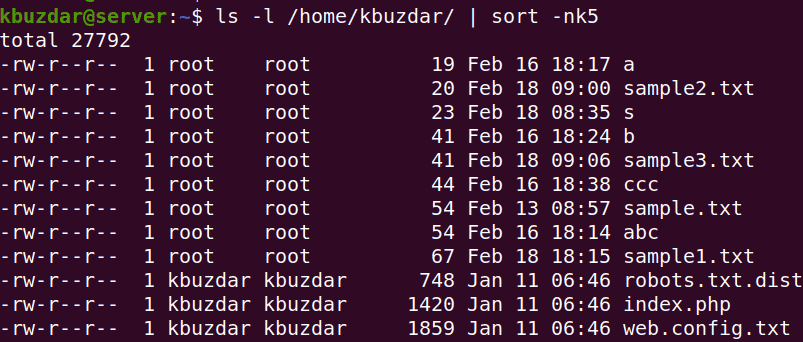In this post, we explained the basic syntax and usage of the sort command in Linux. We also explained some of the common options to extend its functionality. To view help and learn about more sort options, visit the sort man page or type sort –help in Terminal.